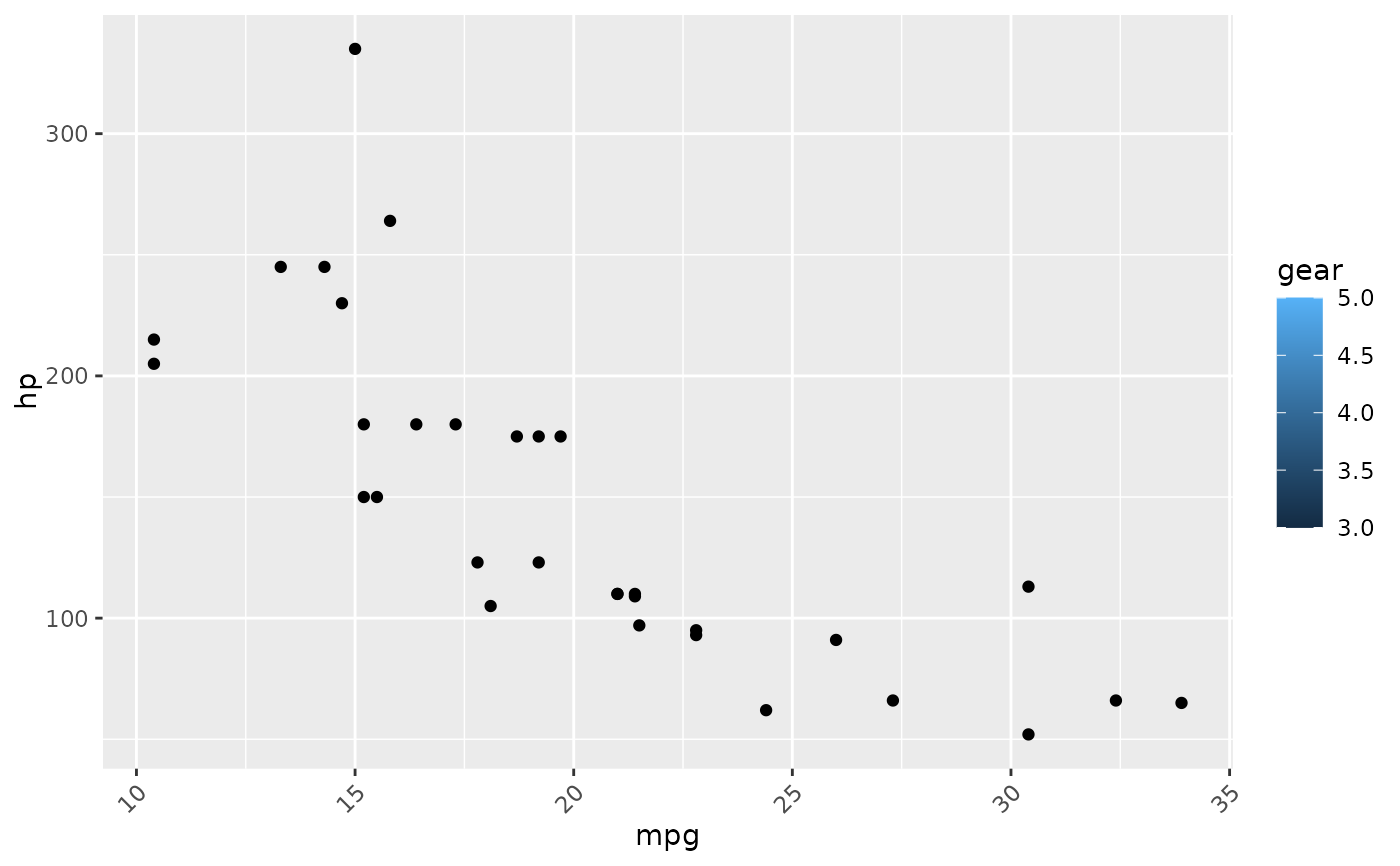Change any of the text parameters such as

easy_change_text(
which = .all_theme_els,
what = .all_element_text,
to = NULL,
teach = FALSE
)

.all_theme_els

.all_element_text

easy_all_text_size(size = NULL, teach = FALSE)

easy_all_text_color(color = NULL, teach = FALSE)

easy_all_text_colour(colour = NULL, teach = FALSE)

easy_text_size(which = .all_theme_els, size = NULL, teach = FALSE)

easy_text_color(which = .all_theme_els, color = NULL, teach = FALSE)

easy_text_colour(which = .all_theme_els, colour = NULL, teach = FALSE)

easy_x_axis_title_size(size, teach = FALSE)

easy_y_axis_title_size(size, teach = FALSE)

easy_x_axis_labels_size(size = NULL, teach = FALSE)

easy_y_axis_labels_size(size = NULL, teach = FALSE)

easy_plot_title_size(size = NULL, teach = FALSE)

easy_plot_subtitle_size(size = NULL, teach = FALSE)

easy_plot_caption_size(size = NULL, teach = FALSE)

easy_plot_legend_size(size = NULL, teach = FALSE)

easy_plot_legend_title_size(size = NULL, teach = FALSE)

easy_center_title(teach = FALSE)

## Arguments

which which element to change (see ggeasy::.all_theme_els) what attribute of the element to change (see ggeasy::.all_element_text) value to which the attribute should be set print longer equivalent ggplot2 expression? size to set attributes to colo(u)r to set attributes to colo(u)r to set attributes to

## Format

An object of class character of length 15.

## Value

a theme object which can be used in ggplot2 calls

Jonathan Carroll

## Examples


library(ggplot2)

# make all text larger
ggplot(mtcars, aes(mpg, hp)) +
geom_point() +
easy_all_text_size(22)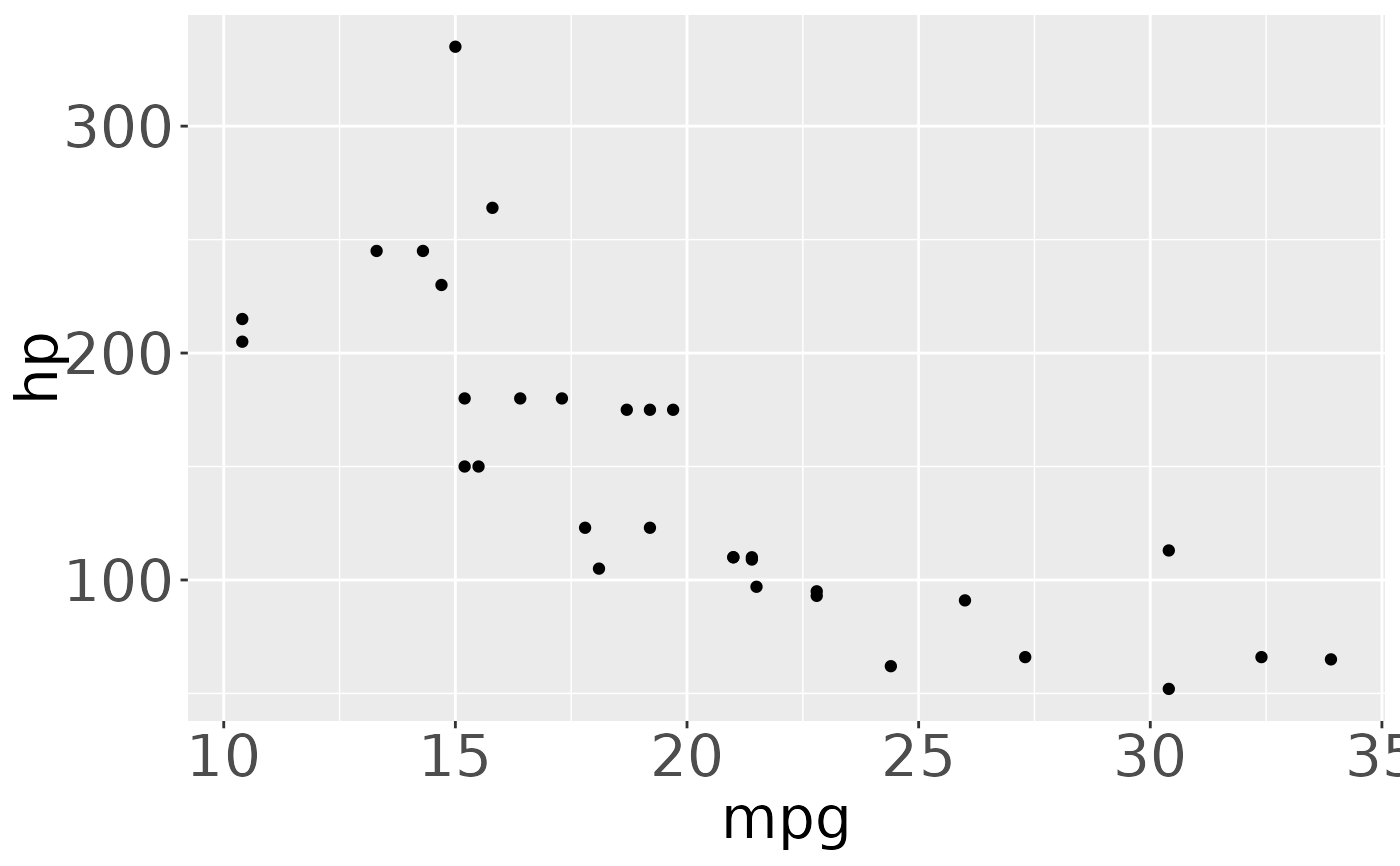# also works if accidentally using easy_text_size(n)

# make the x and y axis text larger
ggplot(mtcars, aes(mpg, hp)) +
geom_point() +
easy_text_size(c("axis.text.x", "axis.text.y"), 22)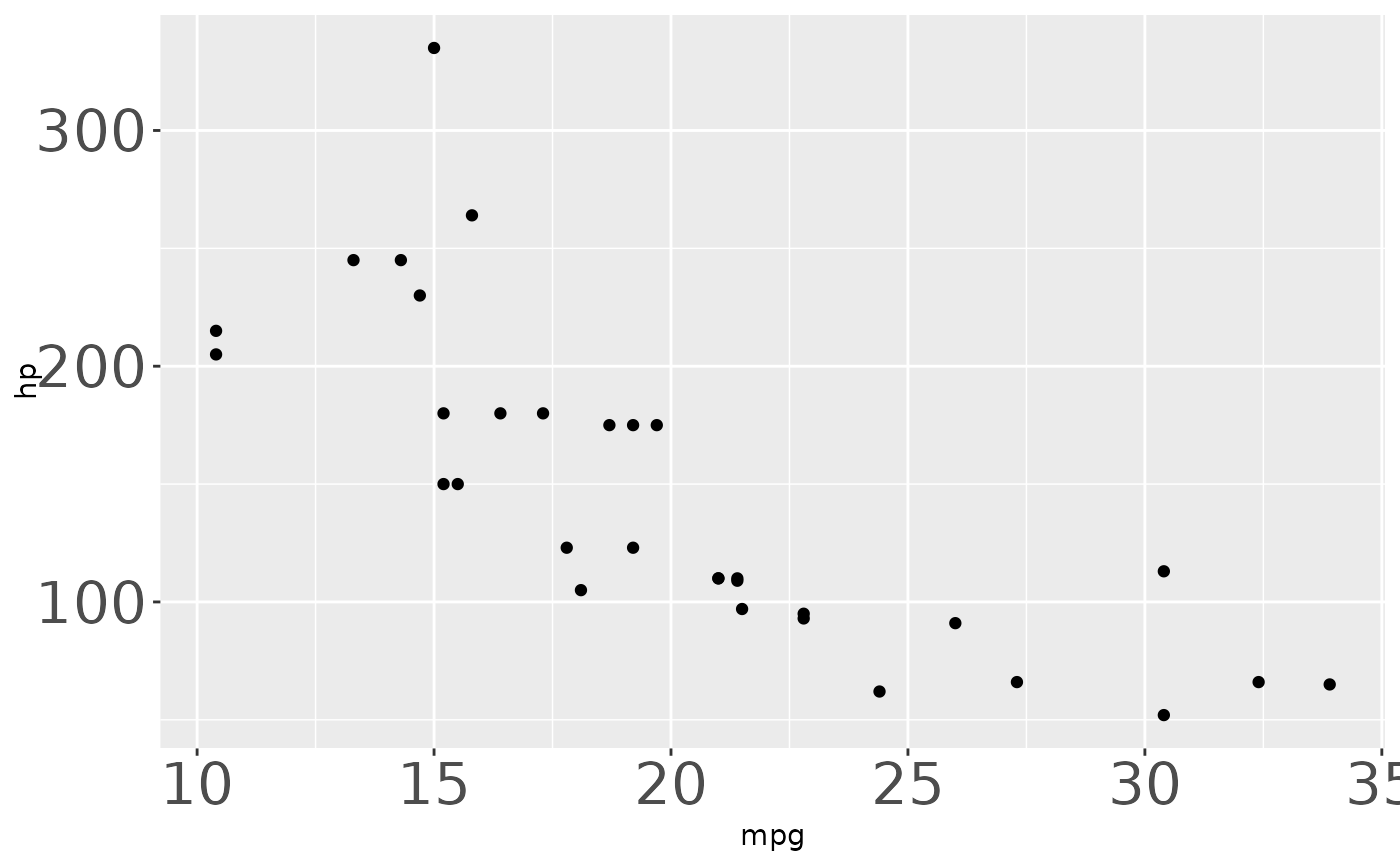# make the x axis labels larger
ggplot(mtcars, aes(mpg, hp)) +
geom_point() +
easy_x_axis_labels_size(22)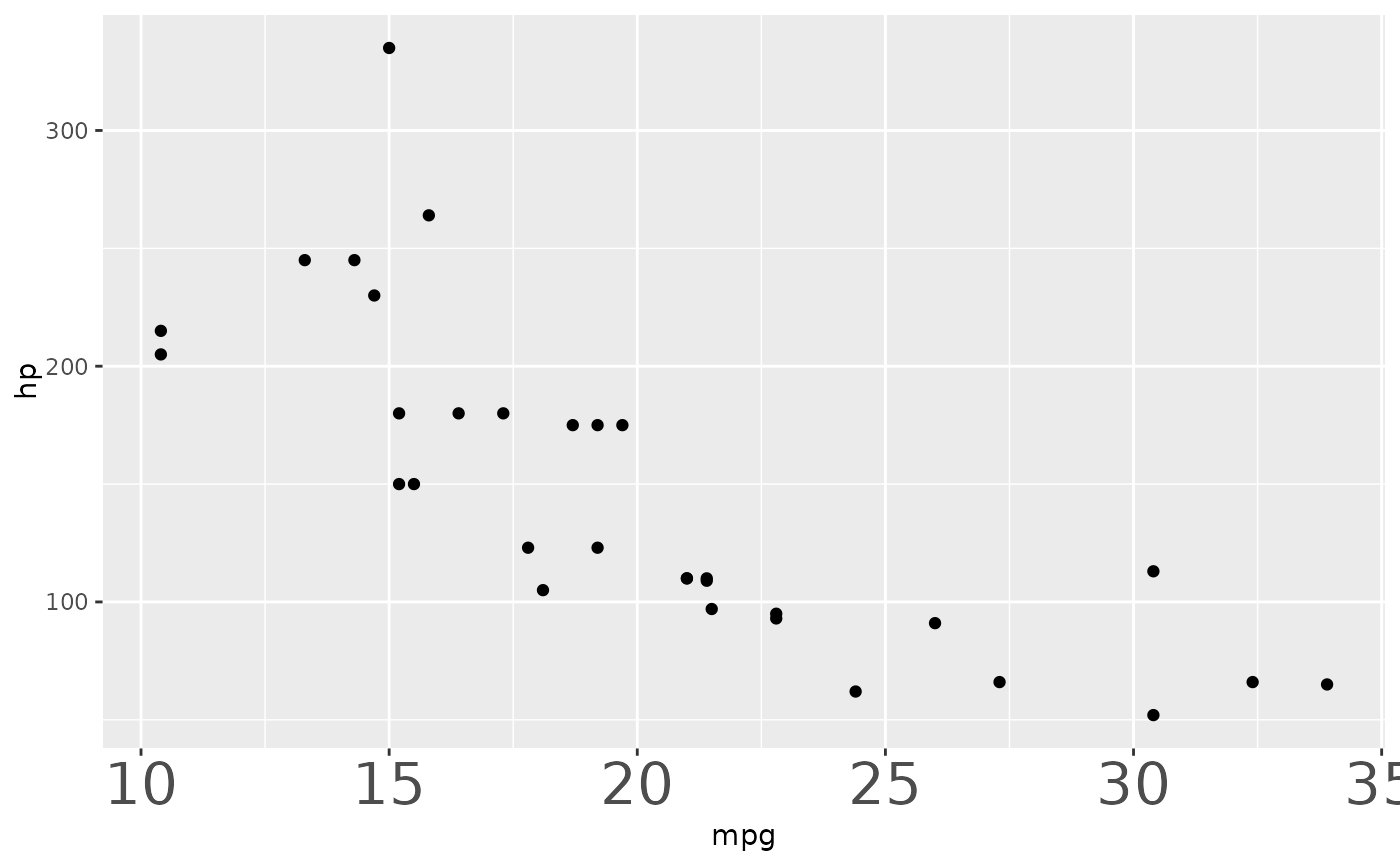# make the plot title larger
ggplot(mtcars, aes(mpg, hp)) +
geom_point() +
labs(title = "My Plot") +
easy_plot_title_size(22)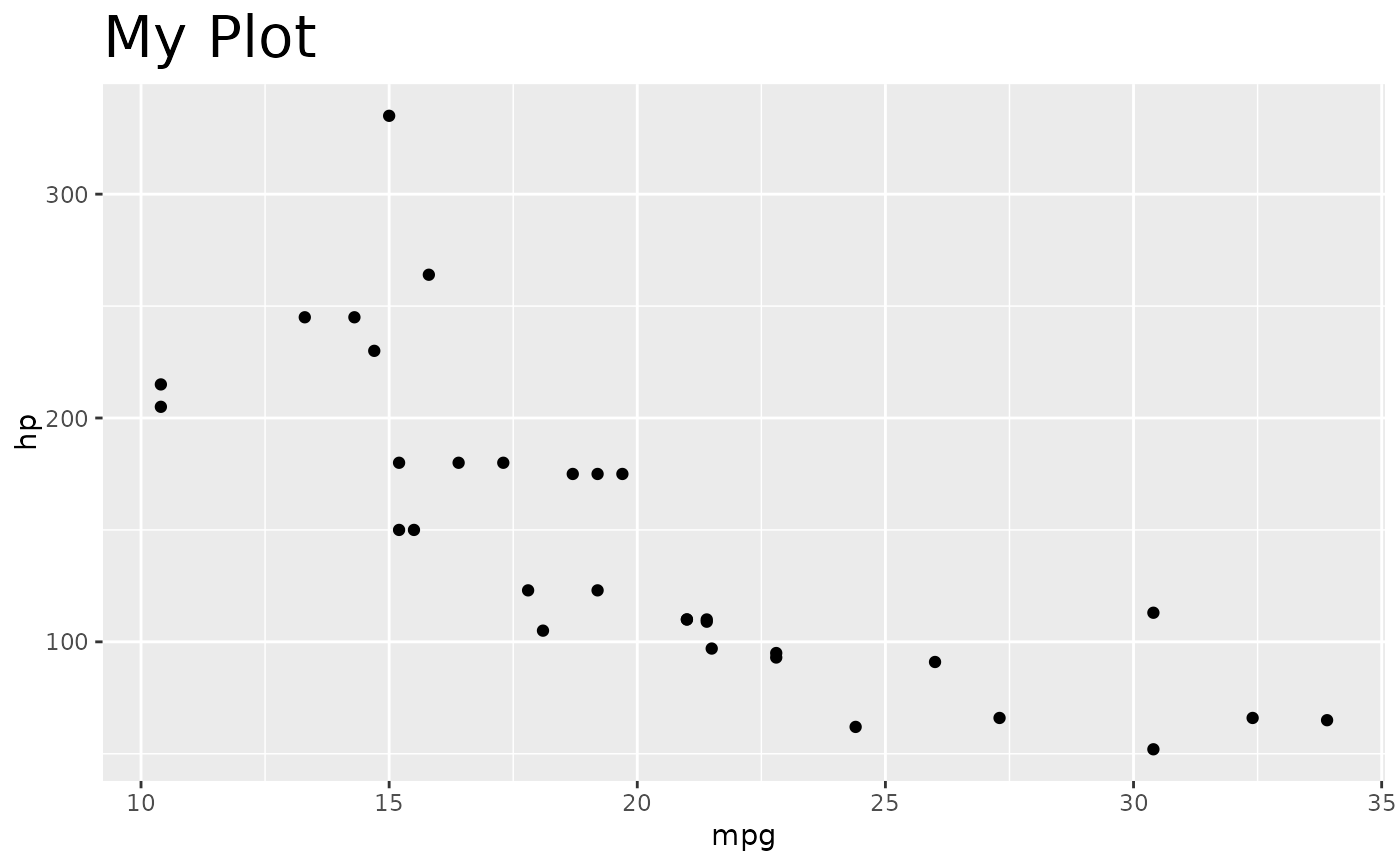# make the legend title larger
ggplot(mtcars, aes(mpg, hp)) +
geom_point(aes(fill = gear)) +
easy_plot_legend_title_size(22)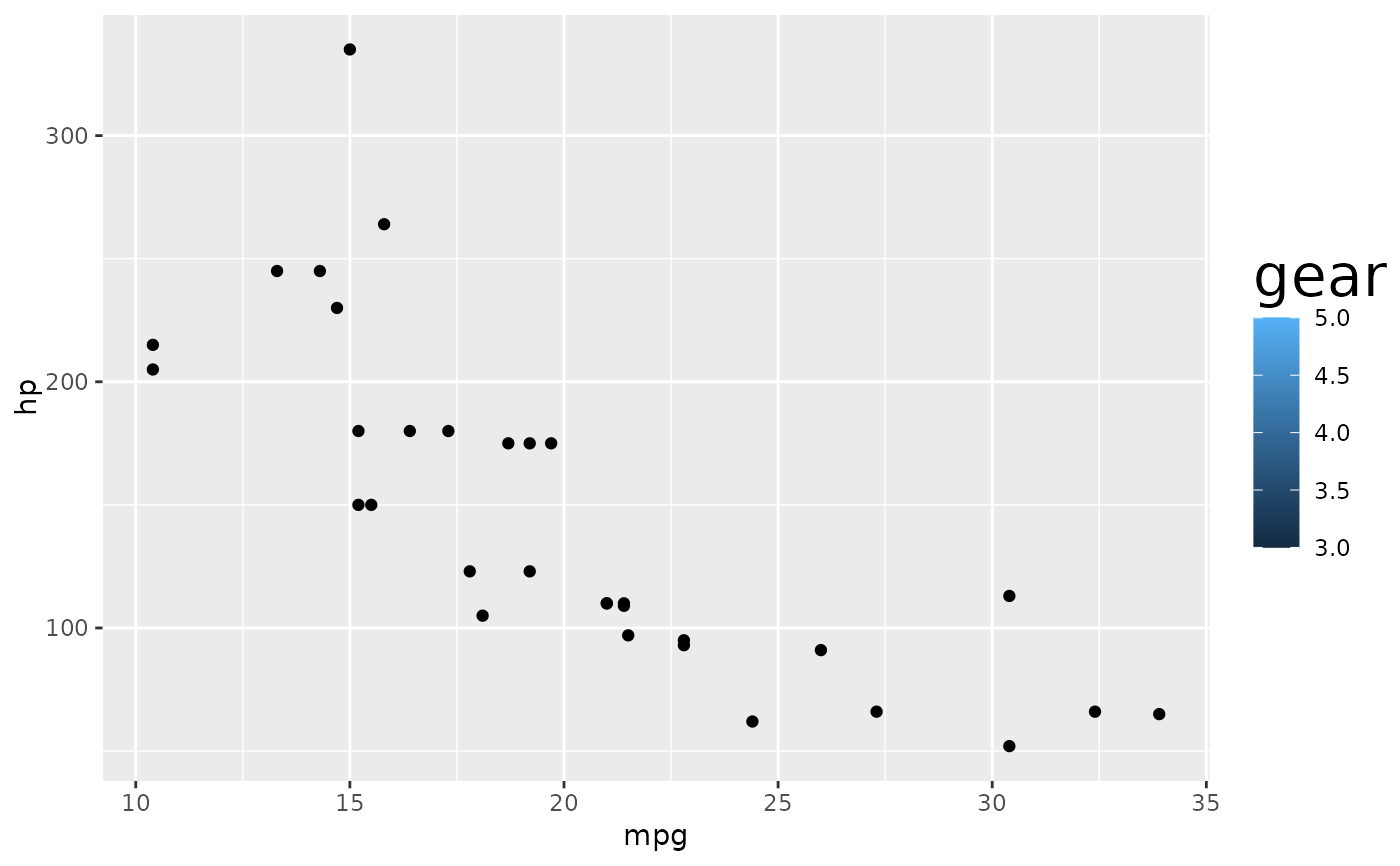# make all the text red
ggplot(mtcars, aes(mpg, hp)) +
geom_point(aes(fill = gear)) +
easy_all_text_color("red")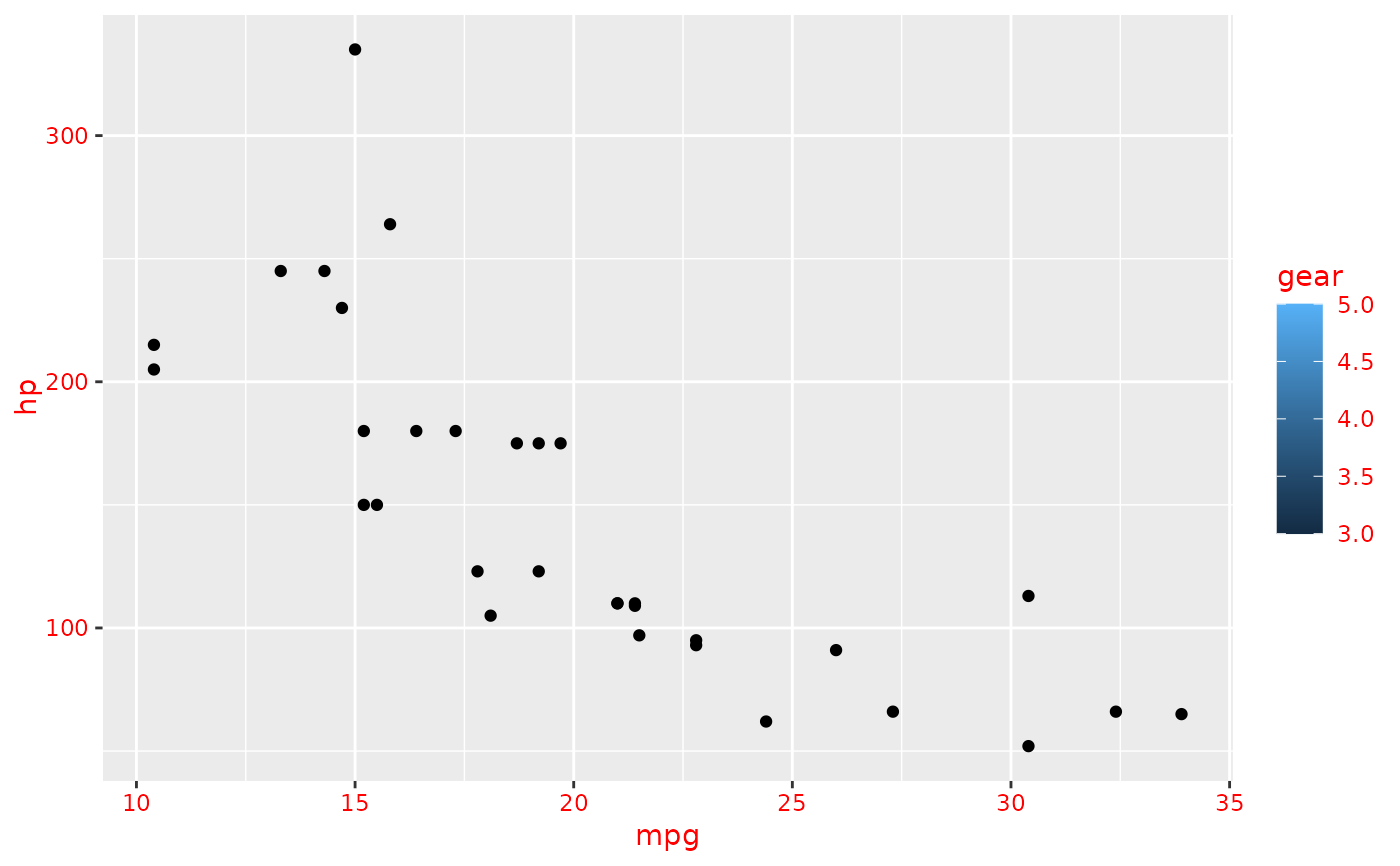# make all the text 45 degrees, right-justified
ggplot(mtcars, aes(mpg, hp)) +
geom_point(aes(fill = gear)) +
easy_change_text(what = "angle", to = 45) +
easy_change_text(what = "hjust", to = 1)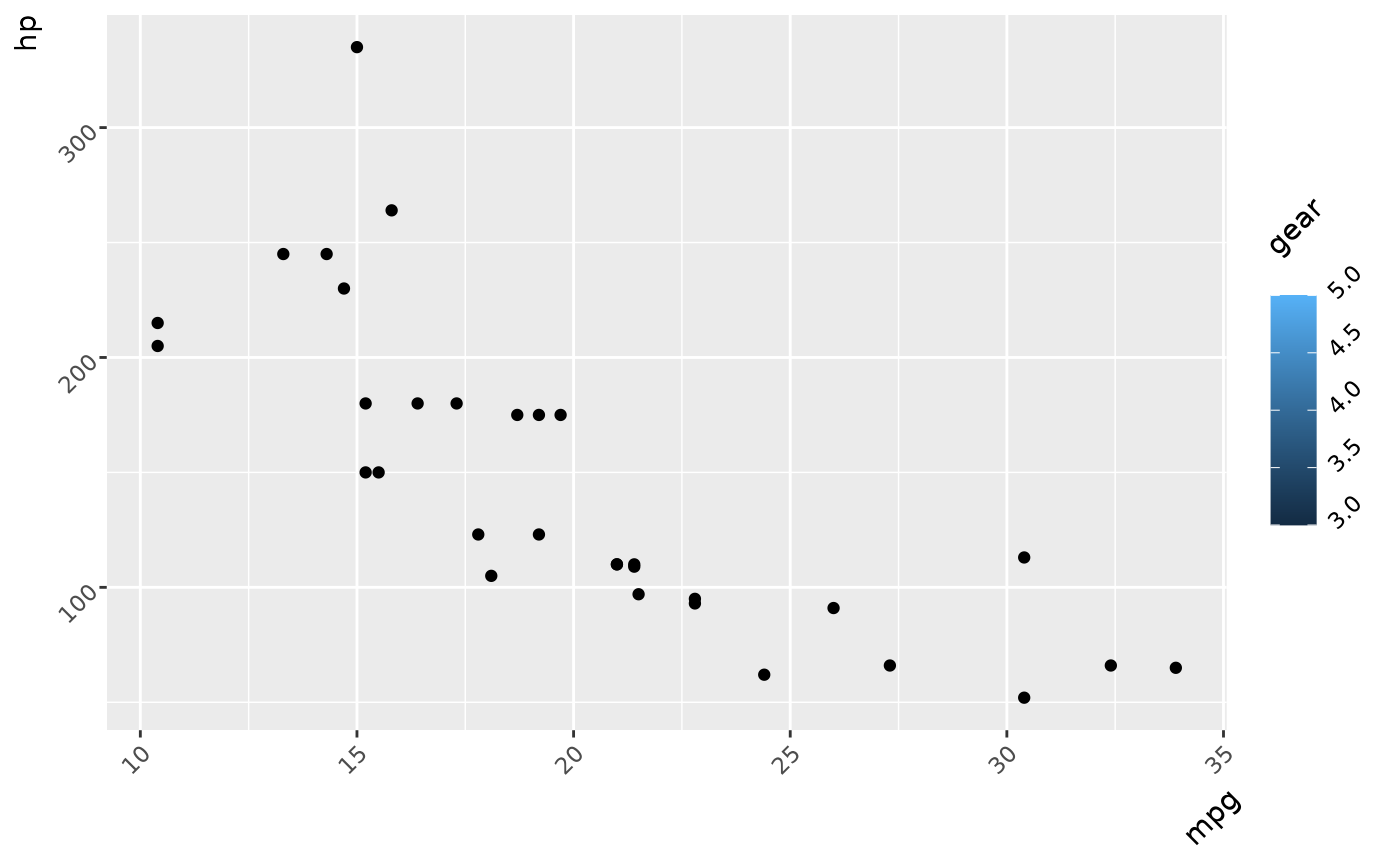# make just x-axis text 45 degrees, right-justified
ggplot(mtcars, aes(mpg, hp)) +
geom_point(aes(fill = gear)) +
easy_change_text(which = "axis.text.x", what = "angle", to = 45) +
easy_change_text(which = "axis.text.x", what = "hjust", to = 1)